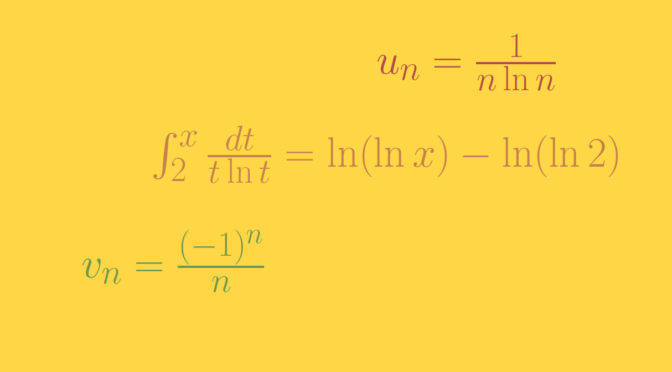# Counterexamples around series (part 1)

Unless otherwise stated, $$(u_n)_{n \in \mathbb{N}}$$ and $$(v_n)_{n \in \mathbb{N}}$$ are two real sequences.

### If $$(u_n)$$ is non-increasing and converges to zero then $$\sum u_n$$ converges?

Is not true. A famous counterexample is the harmonic series $$\sum \frac{1}{n}$$ which doesn’t converge as $\displaystyle \sum_{k=p+1}^{2p} \frac{1}{k} \ge \sum_{k=p+1}^{2p} \frac{1}{2p} = 1/2,$ for all $$p \in \mathbb N$$.

### If $$u_n = o(1/n)$$ then $$\sum u_n$$ converges?

Does not hold as can be seen considering $$u_n=\frac{1}{n \ln n}$$ for $$n \ge 2$$. Indeed $$\int_2^x \frac{dt}{t \ln t} = \ln(\ln x) – \ln (\ln 2)$$ and therefore $$\int_2^\infty \frac{dt}{t \ln t}$$ diverges. We conclude that $$\sum \frac{1}{n \ln n}$$ diverges using the integral test. However $$n u_n = \frac{1}{\ln n}$$ converges to zero.

### If $$u_n = o(v_n)$$ and $$\sum v_n$$ converges then $$\sum u_n$$ converges?

Is not true as we can see taking $$v_n = \frac{(-1)^n}{n}$$ and $$u_n=\frac{1}{n \ln n}$$. $$\sum v_n$$ converges according to the alternating series test and $$\sum u_n$$ diverges according to previous paragraph. However $\lim\limits_{n \to \infty} \left\vert \frac{u_n}{v_n} \right\vert = \lim\limits_{n \to \infty} \frac{1}{\ln n} = 0.$

### If $$\sum u_n$$ is a positive converging series then $$\frac{u_{n+1}}{u_n}$$ is bounded?

Not either. Have a look at $u_n=\begin{cases} \frac{1}{n^2} & \text{for } n \text{ even} \\ \frac{1}{2^n} & \text{for } n \text{ odd.} \end{cases}$ Then for $$p \in \mathbb N$$ $\frac{u_{2p+1}}{u_{2p}} = \frac{2^{2p+1}}{4p^2}$ diverges to $$\infty$$. However $$\sum u_n$$ converges as $$\sum \frac{1}{n^2}$$ and $$\sum \frac{1}{2^n}$$ both converge.